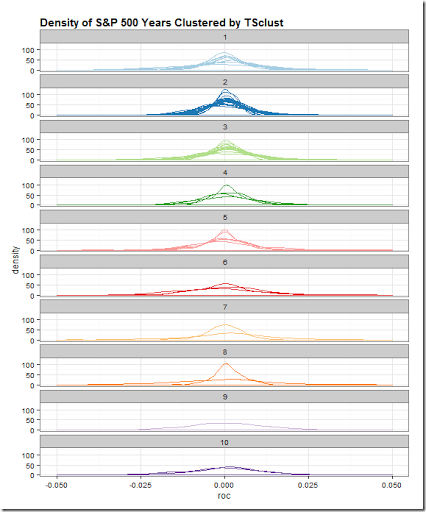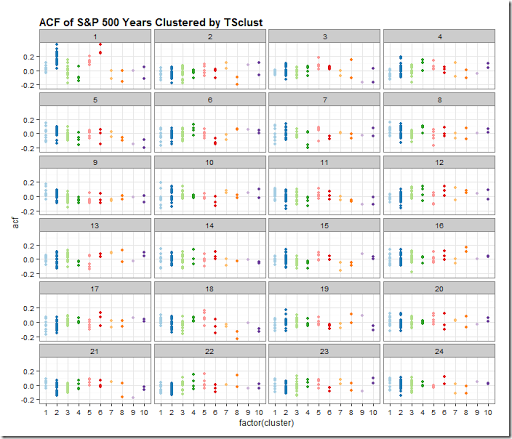Wednesday, March 11, 2015

Extracting Heatmap

Inspired by this tweet, I wanted to try to do something similar in JavaScript.

Fortunately, I had this old post Chart from R + Color from Javascript to serve as a reference, and I got lots of help from these links.

In a couple of hours, I got this crude but working rendering complete with a d3.js brush to get the scale.  Then since this is sort of a finance blog, I imagined we found an old correlation heatmap like the one in Pretty Correlation Map of PIMCO Funds.  Although, we could guess at the correlation values, I thought it would be a lot more fun to get live values.  Try it out below.

1. Brush over the scale / legend
2. Input scale min and max
3. Mouseover color areas in the chart

As I said, it is rough, but it works. It needs a little UI work :)

Thursday, March 5, 2015

Is Time Series Clustering Meaningless? (lots of dplyr)

A kind reader directed me in a comment on Experiments in Time Series Clustering to this paper.

Clustering of Time Series Subsequences is Meaningless: Implications for Previous and Future Research

Eamonn Keogh  and Jessica Lin

Computer Science & Engineering Department University of California – Riverside

http://www.cs.ucr.edu/~eamonn/meaningless.pdf

As I said in my last post, I don’t know what I’m doing, so I have no basis for discussing or arguing time series clustering.  After reading the paper a couple of times, I think I understand their points, and I do not think what I am doing is “meaningless”.  In their financial time series examples, they use prices and speak of trying to find patterns.  I simply want to classify which years are most alike by various characteristics, such as autocorrelation of returns  not prices, distribution of returns, and all sorts of other classifiers.

More than anything this whole exercise gave me a good excuse to dig much, much deeper.  Iongtime readers might be wondering where are the interactive plots.  I wanted to share what I have done so far hoping that readers might elaborate, argue, or point me in good directions.

Regardless of your interest in time series clustering, you might enjoy the dplyr and piping that I used to generate the results.  Also, I have not seen dplyr do applied to autocorrelation ACF, so you might want to check that out in the last snippet of code.

All of the code for this post and last post is in this Github repo.library(TSclust)
library(quantmod)
library(dplyr)
library(pipeR)
library(tidyr)

sp5 <- getSymbols("^GSPC",auto.assign=F,from="1900-01-01")[,4]

sp5 %>>%
# dplyr doesn't like xts, so make a data.frame
(
data.frame(
date = index(.)
,price = .[,1,drop=T]
)
) %>>%
# add a column for Year
mutate( year = as.numeric(format(date,"%Y"))) %>>%
# group by our new Year column
group_by( year ) %>>%
# within each year, find what day in the year so we can join
mutate( pos = rank(date) ) %>>%
mutate( roc = price/lag(price,k=1) - 1 ) %>>%
# can remove date
select( -c(date,price) ) %>>%
as.data.frame %>>%
# years as columns as pos as row
# remove last year since assume not complete
( .[,-ncol(.)] ) %>>%
# remove pos since index will be same
select( -pos ) %>>%
# fill nas with previous value
na.fill( 0 ) %>>%
t %>>%
(~sp_wide) %>>%
# use TSclust diss; notes lots of METHOD options
diss( METHOD="ACF" ) %>>%
hclust %>>%
(~hc) %>>%
ape::as.phylo() %>>%
treewidget #%>>%
#htmlwidgets::as.iframe(file="index.html",selfcontained=F,libdir = "./lib")

library(lattice)
library(ggplot2)
# get wide to long the hard way
# could have easily changed to above pipe to save long
# as an intermediate step
# but this makes for a fun lapply
# and also we can add in our cluster here
sp_wide %>>%
(
lapply(
rownames(.)
,function(yr){
data.frame(
year = as.Date(paste0(yr,"-01-01"),"%Y-%m-%d")
,cluster = cutree(hc,10)[yr]
,pos = 1:length(.[yr,])
,roc = .[yr,]
)
}
)
) %>>%
(do.call(rbind,.)) %>>%
(~sp_long)

sp_long %>>%
ggplot( aes( x = roc, group = year, color = factor(cluster) ) ) %>>%
+ geom_density() %>>%
+ facet_wrap( ~ cluster, ncol = 1 ) %>>%
+ xlim(-0.05,0.05) %>>%
+ labs(title='Density of S&P 500 Years Clustered by TSclust') %>>%
+ theme_bw() %>>%
# thanks to my friend Zev Ross for his cheatsheet
+ theme( plot.title = element_text(size=15, face="bold", hjust=0) ) %>>%
+ theme( legend.position="none" ) %>>%
+ scale_color_brewer( palette="Paired" )# explore autocorrelations
sp5 %>>%
# dplyr doesn't like xts, so make a data.frame
(
data.frame(
date = index(.)
,price = .[,1,drop=T]
)
) %>>%
# add a column for Year
mutate( year = as.numeric(format(date,"%Y"))) %>>%
# group by our new Year column
group_by( year ) %>>%
# within each year, find what day in the year so we can join
mutate( pos = rank(date) ) %>>%
mutate( roc = price/lag(price,k=1) - 1 ) %>>%
# can remove date
select( -c(date,price) ) %>>%
as.data.frame %>>%
# years as columns as pos as row
# remove last year since assume not complete
( .[,-ncol(.)] ) %>>% t -> sP

sp_long %>>%
group_by( cluster, year ) %>>%
do(
. %>>%
(
clustd ~
acf(clustd\$roc,plot=F) %>>%
(a ~
data.frame(
cluster = clustd[1,2]
,year = clustd[1,1]
,lag = a\$lag[-1]
,acf = a\$acf[-1]
)
)
)
) %>>%
as.data.frame %>>%
ggplot( aes( x = factor(cluster), y = acf, color = factor(cluster) ) ) %>>%
+ geom_point() %>>%
+ facet_wrap( ~lag, ncol = 4 ) %>>%
+ labs(title='ACF of S&P 500 Years Clustered by TSclust') %>>%
+ theme_bw() %>>%
# thanks to my friend Zev Ross for his cheatsheet
+ theme(
plot.title = element_text(size=15, face="bold", hjust=0)
,legend.title=element_blank()
) %>>%
+ theme(legend.position="none") %>>%
+ scale_color_brewer(palette="Paired")

If you’ve made it this far, I would love to hear from you.

Monday, March 2, 2015

Experiments in Time Series Clustering

Last night I spotted this tweet about the R package TSclust.

I should start by saying that I really don’t know what I’m doing, so be warned.  I thought it would interesting to apply TSclust to the S&P 500 price time series.  I took the 1-day simple rate of change, grouped by year with dplyr, and then indexed by the day of the year all in one pipeR pipeline.  Since the TSclust paper

TSclust: An R Package for Time Series Clustering

Journal of Statistical Software, Volume 62, Issue 1

November 2014

http://www.jstatsoft.org/v62/i01/paper

demonstrates interoperability with hclust in their OECD interest rate example ( Section 5.2 ), I thought I could visualize the results nicely with treewidget from the epiwidgets package.  Just because the htmlwidget was designed for phylogeny doesn’t mean we can’t use it for finance.  Here is the result.

For reference and searching, I’ll copy the code below, but all of this can be found in this Github repo.

library(TSclust) library(quantmod) library(dplyr) library(pipeR) library(tidyr) library(epiwidgets) sp5 <- getSymbols("^GSPC",auto.assign=F,from="1900-01-01")[,4] sp5 %>>% # dplyr doesn't like xts, so make a data.frame ( data.frame( date = index(.) ,price = .[,1,drop=T] ) ) %>>% # add a column for Year mutate( year = as.numeric(format(date,"%Y"))) %>>% # group by our new Year column group_by( year ) %>>% # within each year, find what day in the year so we can join mutate( pos = rank(date) ) %>>% mutate( roc = price/lag(price,k=1) - 1 ) %>>% # can remove date select( -c(date,price) ) %>>% as.data.frame %>>% # years as columns as pos as row spread( year, roc ) %>>% # remove last year since assume not complete ( .[,-ncol(.)] ) %>>% # remove pos since index will be same select( -pos ) %>>% # fill nas with previous value na.fill( 0 ) %>>% t %>>% # use TSclust diss; notes lots of METHOD options diss( METHOD="ACF" ) %>>% hclust %>>% ape::as.phylo() %>>% treewidget